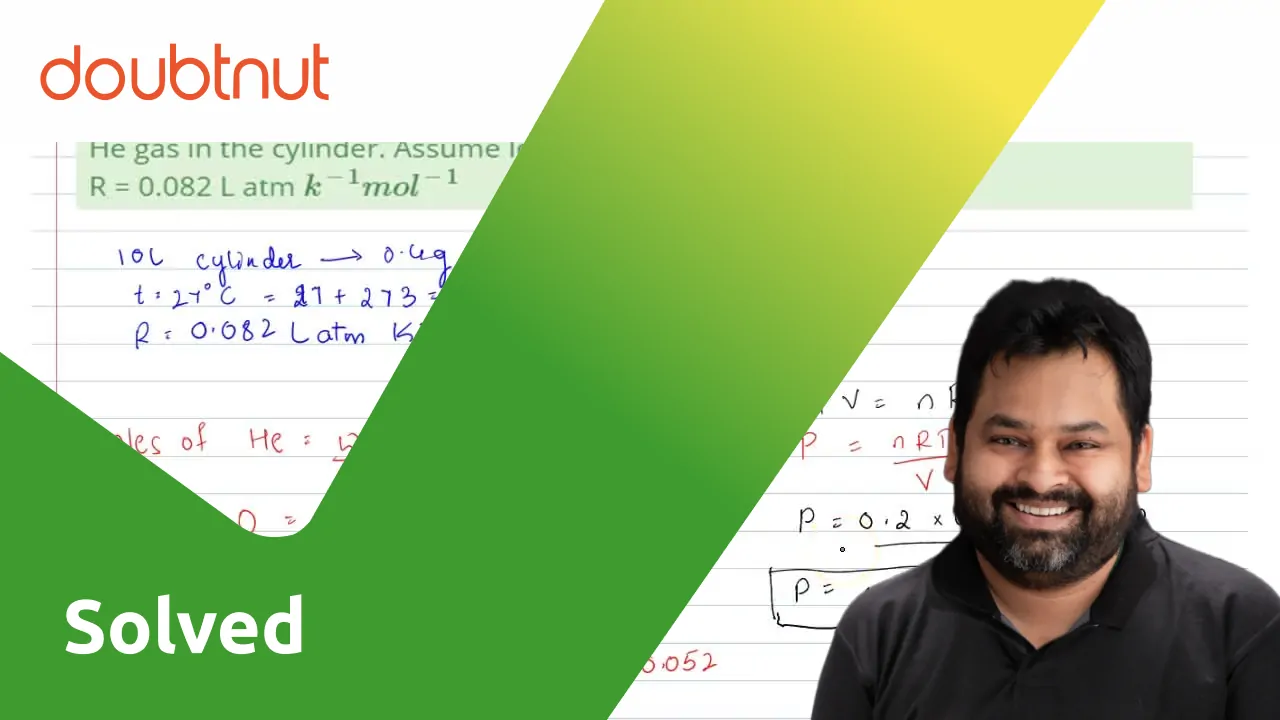Doubtnut App - Get 50 Lakh+ video solutions better than GoogleInstall Now

# Calculate the total pressure in a 10 L cylinder which contains 0.4 g of helium, 1.6 g of oxygen and 1.4 g of nitrogen at 27∘C. Also calculate the partial pressures of He gas in the cylinder. Assume Ideal behaviour for gases. R = 0.082 L atm k−1mol−1Solution in Kannada

Text Solution
Verified by Experts

## ptotal=0.4926 atm pHe=0.2463 atm pO2=0.1231 atm pN2=0.123 atm